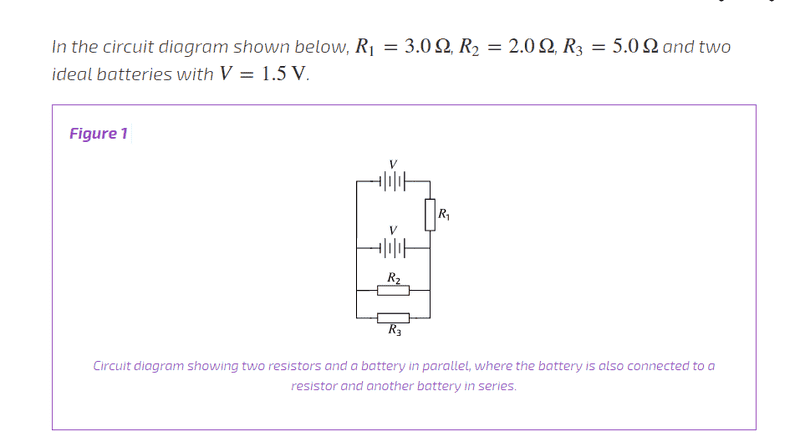Circuit question with two batteries and resistors in pa

Homework Statement

[/B]Q: What is the power dissipated by R1?

Homework Equations

Ohm's law: V = IR
Power: P = VI, I2R, V2/R
Kirchoff's circuit and voltage laws

The Attempt at a Solution

I assumed the currents were I1 travelling rightwards from the top battery, I2 rightwards from the second battery, and came together to make I3 for the bottom branch. The bottom resistor pair I simplified to 10/7 Ohms. From here I assume I have to set up simultaneous equations to solve for I1.

I used the equation for the bottom branch 1.5V - 10/7 * I3 = 0 to get I3 = 1.05A.

I subbed this into the equation for the outer loop 1.5 - 3I1 - 10/7I3 = 0. However plugging 1.05A into this gave me 0 for I1...

I feel like I'm way off the mark..

•